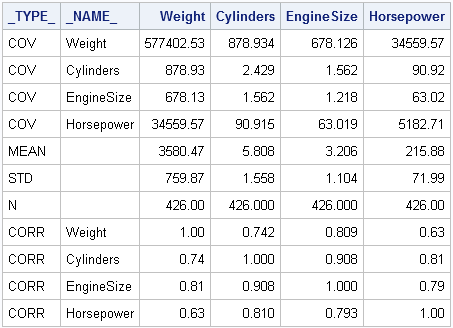SAS has several kinds of special data sets whose contents are organized according to certain conventions. These special data sets are marked with the TYPE= data set attribute.

For example, the CORR procedure can create a data set with the TYPE=CORR attribute. You can decipher the structure of the data set by using the _TYPE_ variable. For example, the following PROC CORR statement creates a TYPE=CORR data set:

```/* create TYPE=CORR data set */ proc corr data=sashelp.cars out=Corr cov nomiss; var Weight Cylinders EngineSize HorsePower; quit;   proc print data=Corr noobs; run;```The covariance matrix is contained in the observations for which _TYPE_="COV". Similarly, the correlation matrix is contained in the observations for which _TYPE_="CORR". The observation for which _TYPE_="MEAN" contains the sample means, _TYPE_="STD" contains the standard deviations, and so on. Another special variable, _NAME_, identifies the rows of the covariance and correlation matrices.

How can you read a special SAS data set into matrices in the SAS/IML language? There are several ways to do this, but a useful technique is to use a WHERE clause on the READ statement to subset the observations, as follows:

```/* Read special SAS data set into SAS/IML matrices and vectors */ proc iml; use Corr; read all var _num_ where(_TYPE_="COV") into cov[r=_NAME_ c=VarNames]; read all var _num_ where(_TYPE_="CORR") into corr; read all var _num_ where(_TYPE_="MEAN") into mean; read all var _num_ where(_TYPE_="STD" ) into std; close Corr;   print cov, mean, std, corr;```Notice several points:

• The _NUM_ keyword reads all numerical variables. Therefore the same code works for any TYPE=CORR data set, not just the one in this example.
• The WHERE clause is used to select certain observations within this structured data set. Although the WHERE statements cause the data set to be read multiple times, reading the data is usually cheap compared with the computational cost of the rest of a SAS/IML program.
• The R= and C= options on the first READ statement are abbreviations for the ROWNAME= and COLNAME= options. These options do two things. First, they create two vectors, _NAME_ and VarNames, that contain the names of all numerical variables in the data set. Second, they implicitly call the MATTRIB statement to assign rown ames and column names to the cov matrix. Notice that the PRINT statement automatically printed the column and row headings for the cov matrix.

You can use this trick to read any kind of a structured data set into SAS/IML matrices. It is not always the most efficient way to read the data, but it is very general and can be used to read the "special" TYPE= data sets that are created by SAS procedures.

Share